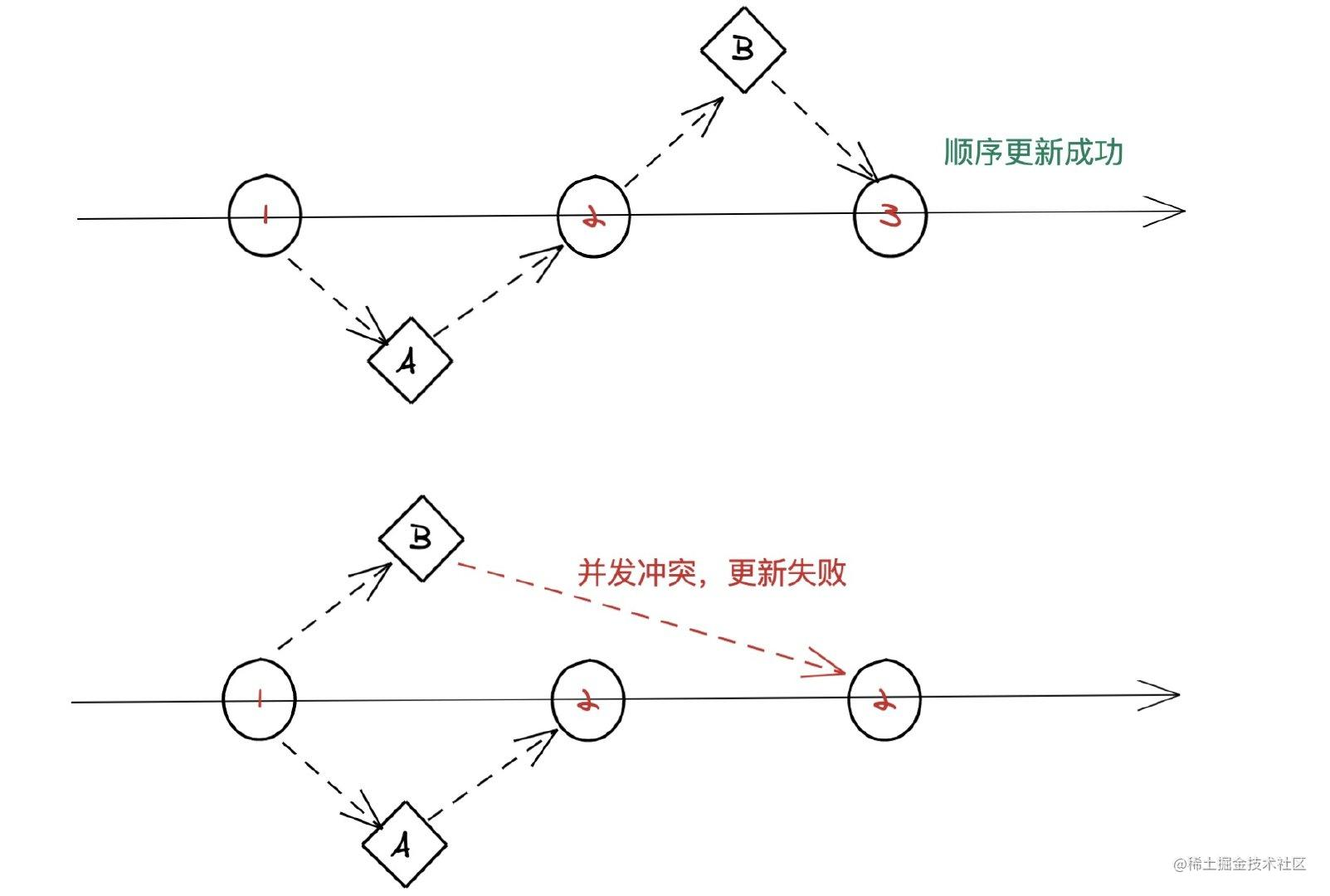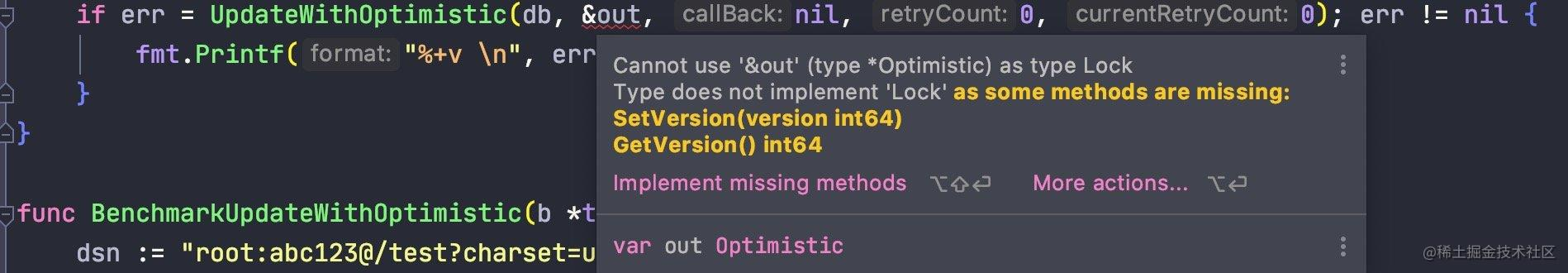# 写了一个 gorm 乐观锁插件# 实现

``````UPDATE `table` SET `amount`=100,`version`=version+1 WHERE `version` = 1 AND `id` = 1
````````````type Optimistic struct {
Id      int64   `gorm:"column:id;primary_key;AUTO_INCREMENT" json:"id"`
UserId  string  `gorm:"column:user_id;default:0;NOT NULL" json:"user_id"` // 用户ID
Amount  float32 `gorm:"column:amount;NOT NULL" json:"amount"`             // 金额
Version int64   `gorm:"column:version;default:0;NOT NULL" json:"version"` // 版本
}

func TestUpdate(t *testing.T) {
dsn := "root:abc123@/test?charset=utf8&parseTime=True&loc=Local"
db, err := gorm.Open(mysql.Open(dsn), &gorm.Config{})
var out Optimistic
db.First(&out, Optimistic{Id: 1})
out.Amount = out.Amount + 10
column := db.Model(&out).Where("id", out.Id).Where("version", out.Version).
UpdateColumn("amount", out.Amount).
UpdateColumn("version", gorm.Expr("version+1"))
fmt.Printf("#######update %v line \n", column.RowsAffected)
}
``````

``````
var out Optimistic
db.First(&out, Optimistic{Id: 1})
out.Amount = out.Amount + 10
if err = UpdateWithOptimistic(db, &out, nil, 0, 0); err != nil {
fmt.Printf("%+v \n", err)
}
``````
• 这里的使用场景是每次更新时将 `amount` 金额加上 `10`

``````func BenchmarkUpdateWithOptimistic(b *testing.B) {
dsn := "root:abc123@/test?charset=utf8&parseTime=True&loc=Local"
db, err := gorm.Open(mysql.Open(dsn), &gorm.Config{})
if err != nil {
fmt.Println(err)
return
}
b.RunParallel(func(pb *testing.PB) {
var out Optimistic
db.First(&out, Optimistic{Id: 1})
out.Amount = out.Amount + 10
err = UpdateWithOptimistic(db, &out, func(model Lock) Lock {
bizModel := model.(*Optimistic)
bizModel.Amount = bizModel.Amount + 10
return bizModel
}, 3, 0)
if err != nil {
fmt.Printf("%+v \n", err)
}
})
}
``````

`amount` 金额 `+10`，失败时再次依然将金额+10，尝试更新 `3` 次；经过上述的并行测试，最终查看数据库确认数据并没有发生错误。

## 面向接口编程

``````func UpdateWithOptimistic(db *gorm.DB, model Lock, callBack func(model Lock) Lock, retryCount, currentRetryCount int32) (err error) {
if currentRetryCount > retryCount {
return errors.WithStack(NewOptimisticError("Maximum number of retries exceeded:" + strconv.Itoa(int(retryCount))))
}
currentVersion := model.GetVersion()
model.SetVersion(currentVersion + 1)
column := db.Model(model).Where("version", currentVersion).UpdateColumns(model)
affected := column.RowsAffected
if affected == 0 {
if callBack == nil && retryCount == 0 {
return errors.WithStack(NewOptimisticError("Concurrent optimistic update error"))
}
time.Sleep(100 * time.Millisecond)
db.First(model)
bizModel := callBack(model)
currentRetryCount++
err := UpdateWithOptimistic(db, bizModel, callBack, retryCount, currentRetryCount)
if err != nil {
return err
}
}
return column.Error

}
``````

• 判断重试次数是否达到上限。
• 获取当前更新对象的版本号，将当前版本号 +1。
• 根据版本号条件执行更新语句。
• 更新成功直接返回。
• 更新失败 `affected == 0` 时，执行重试逻辑。
• 重新查询该对象的最新数据，目的是获取最新版本号。
• 执行回调函数。
• 从回调函数中拿到最新的业务数据。
• 递归调用自己执行更新，直到重试次数达到上限。

``````type Lock interface {
SetVersion(version int64)
GetVersion() int64
}
``````

``````func (o *Optimistic) GetVersion() int64 {
return o.Version
}

func (o *Optimistic) SetVersion(version int64) {
o.Version = version
}
````````````currentVersion := model.GetVersion()
model.SetVersion(currentVersion + 1)
``````

## 类型断言

``````err = UpdateWithOptimistic(db, &out, func(model Lock) Lock {
bizModel := model.(*Optimistic)
bizModel.Amount = bizModel.Amount + 10
return bizModel
}, 2, 0)
if err != nil {
fmt.Printf("%+v \n", err)
}
``````

`Go` 语言中这样的行为被称为`类型断言`；虽然叫法不同，但目的类似。其语法如下：

``````x.(T)
x:表示 interface
T:表示 向下转型的具体 struct
``````

# 总结

github.com/crossoverJi…